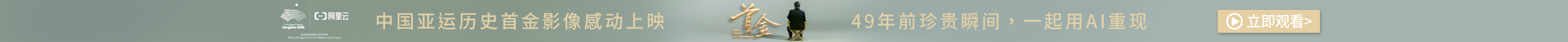## C++字符串的使用

String类的构造函数和析构函数如下：

a) string s; //生成一个空字符串s

b) string s(str) //拷贝构造函数 生成str的复制品

c) string s(str,stridx) //将字符串str内“始于位置stridx”的部分当作字符串的初值

d) string s(str,stridx,strlen) //将字符串str内“始于stridx且长度顶多strlen”的部分作为字符串的初值

e) string s(cstr) //将C字符串作为s的初值

f) string s(chars,chars_len) //将C字符串前chars_len个字符作为字符串s的初值。

g) string s(num,c) //生成一个字符串，包含num个c字符

h) string s(beg,end) //以区间beg;end(不包含end)内的字符作为字符串s的初值

i) s.~string() //销毁所有字符，释放内存

1)    =,assign() //赋以新值

2)    swap() //交换两个字符串的内容

3)     +=,append(),push_back() *//在尾部添加字符*

4)    insert() //插入字符**

5)    erase() //删除字符**

6)     clear() //删除全部字符**

7)    replace() //替换字符**

8)    //串联字符串**

9)     ==,!=,<,<=,>,>=,compare() //比较字符串

10)  size(),length() //返回字符数量

11) max_size() //返回字符的可能最大个数

12) empty() //判断字符串是否为空

13) >>,getline() //从stream读取某值

14) copy() //将某值赋值为一个C_string

15) substr() //返回某个子字符串

16) 查找函数  find()

posted on 2019-05-04 20:17  Mr-Miller  阅读(292)  评论(0编辑  收藏  举报